## strange result from select....

I can't understand why select(has,3*C,x) returns 1  and select(has,3+C,x) returns 0

I was expecting to get NULL or {}. But when doing select(has,[3*C],x) and select(has,[3+C],x)  now both return [ ] as expected

Where did 1 and 0 come from in the above examples? I looked at help page, but do not see it.

restart;
select(has,3*C,x)

restart;
select(has,3+C,x)

or the same:

restart;
select(z->has(z,x),3*C);
select(z->has(z,x),3+C)



The select function selects the operands of the expression e which satisfy
the Boolean-valued procedure f, and creates a new object of the same type
as e. Those operands for which f does not return true are discarded in the
newly created object.

The above is the same as if one typed

select(x->false,3+C);
0

select(x->false,3*C);
1


## how to use indets with conditions?...

I am trying to learn how do somethings without using pattern matching and it is a struggle so far for me.

For an example, now I want to ask Maple to tell me if C (which is a constant) exist in expression, as long as C does not occur as argument to exp(....)

I'll explain why I want to do this and show small example and show how I ended solving it and ask if there is better way.

Given this expression (this can be result of dsolve for example. Made up here)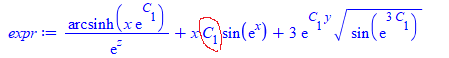I just need to determine if the expression has C anywhere, which is NOT an argument to exp(). In the above example, there is one.

The reason I want to find this, is that this is the constant of integration for first order ODE, and I want to repalce exp(C) by constant C, but as long as there is no C allready anywhere in expression on its, otherwise I need to introduce new constant C, which I do not want to do (since first order, should have only one constant of integration) and in this case will leave the expression as is and not try to simplify it.

But indets tells one that C is there, not where. This is what I tried

restart;
expr:=1/exp(z)*arcsinh(x*exp(C))+x*C*sin(exp(x))+3*exp(C*y)*sqrt(sin(exp(3*C)));
indets(expr, specfunc(exp));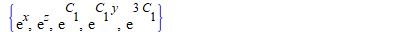Now I can find if C is argument to exp(....) from the above or not by more processing. (using applyrule or subsindets, etc...)

But first I still need to to determine if there is C that exist anywhere else, as long it is not inside exp(.....).  If I do

indets(expr, C);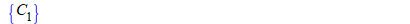But this does not tell me the information I want. It just says expression has C somewhere.

I looked at evalindets and looked at applyrule and do not see how to use these to do what I want.

Basically I want to tell Maple this

indets(expr,C, conditional( C is not anywhere inside exp(.....) ))

I can solve this using pattern matching. But I fnd Maple pattern matching awkaward to use sometimes and trying to learn how to do things without it.

So this is how I ended solving it. I replace all the C inside exp(....) with ZZZ. Then use indets again to check if C still there or not. This tells me what I want.

restart;
expr:=1/exp(z)*arcsinh(x*exp(C))+x*C*sin(exp(x))+3*exp(C*y)*sqrt(sin(exp(3*C)));
new_expr:=subsindets(expr, specfunc(exp), ()-> ZZZ);
if indets(new_expr, C)<>{} then
print("C exist outside exp()");
else
print("C does not exist outside exp()");
fi;


"C exist outside exp()"

And

restart;
expr:=1/exp(z)*arcsinh(x*exp(C))+x*sin(exp(x))+3*exp(C*y)*sqrt(sin(exp(3*C)));
new_expr:=subsindets(expr, specfunc(exp), ()-> ZZZ);
if indets(new_expr, C)<>{} then
print("C exist outside exp()");
else
print("C does not exist outside exp()");
fi;


"C does not exist outside exp()"

is there a better way to do this?

## The fastest way to search the elements of one list...

Hello again

I need to find the position of the elements of one list in another list.  Here it is a simple example

L1:=[837, 526, 283, 216, 93, 512, 161, 202, 76, 851, 503, 437, 147, 36, 922];
L2:=[526, 283, 202, 437, 922, 865, 879, 804, 473, 325];
remove(has,map(x->ListTools:-Search(x,L1),L2),0)



the position of the elements in list L1 is [2, 3, 8, 12, 15].

For huge lists, this solution is not efficient.  Can that be done faster and less memory demanding? (Threads or Grid safe?).

Many thanks.

Ed

## How to Build a Package Module from Collected Piece...

I am creating a largish package (i.e., for use by others with the “with” command).

Most of the exported procedures have some derivations associated with the code.  This is at least a partial reason for using Maple.  So, I had organized a worksheet so that each exported procedure has its own section that included pre-coding derivations, the procedure, and some post-coding validation of the code (serious testing is done elsewhere).  At the end of the worksheet I had a section that built the module and saved it to the appropriate library.  If I execute the worksheet it runs through everything and builds a new version of the library containing the package.

Getting these exported procedures to bind to a local helper procedure that is shared among several exported procedures was a bit tricky.  The way I ended up doing it is illustrated in the attached worksheet.

 >>(1)
 >(2)
 >>>>>>>
 >>(3)
 >(4)
 >(5)
 >## how to replace multiple items in expression withou...

Given some expression, I want to replace each exp(.....) in it with something else.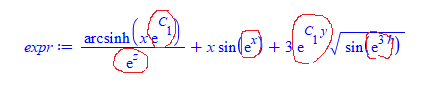This is what I currently do. First I find all the exp() terms, then using a loop and use subs  like this

restart;
expr:=1/exp(z)*arcsinh(x*exp(C))+x*sin(exp(x))+3*exp(C*y)*sqrt(sin(exp(3*h)));

#find all exp terms
s:=select(x->has(x,exp),indets(expr));
s:=select(x->op(0,x)='exp',s)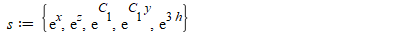Now, lets says I want to replace each with Z

I tried to use but that did not work.

So now I am doing this

for item in s do
expr:=subs(item=Z,expr);
od:
expr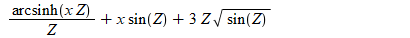is it possible do this without a loop? could not do it using ~

With map, I can do this map(x->subs(x=Z,expr),s) but this does not replace it inside expr where I want.

Assignment is not allowed inside the above so I can not do  this map(x->expr:=subs(x=Z,expr),s)

And if I do expr:=map(x->subs(x=Z,expr),s)  it does not work either. it gives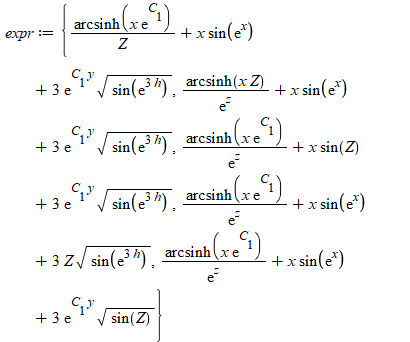How to do the replacement without using a do loop?

## How to plot a graph that depends on an integral....

I have the following question, plot the graph of mu=5*log_10(D_L/10) where D_L=(c/H_0)*\int_0^z dz'/[A(1+z')^4+B(1+z)^3+C]^{1/2} with resepct to z, where A,B,C are numerical values given beforehand, and c is the speed of light and H_0 is the current Hubble constant.

Thanks, just by experience, can I learnt these syntax languages.

Peace out!

## Help with solving ODE?...

Might anyone be able to tell me what I am doing wrong trying to solve for this ODE?

Thanks so much!

## Why inequal plot like this?...

Hi there,

Can someone explain to me why is it happening? If smaller equal than coloured well, if smaller then just the equal line appears. Why?

with(plots);
dm1 := .23; pm12 := .7; dm12 := .23;
inequal(pm1*(dm12+am21)-pm12*(dm1+am21) <= 0, pm1 = 0 .. 1, am21 = 0 .. 1, color = "Nautical 1");inequal(pm1*(dm12+am21)-pm12*(dm1+am21) < 0, pm1 = 0 .. 1, am21 = 0 .. 1, color = "Nautical 1")Thank you!

## This code is not running. kindly help me out for f...

restart;
PDEtools[declare](f(x), prime = x);
N := 4;
f(x) :=  sum(p^i*f[i](x), i = 0..N):
HPMEq := (1 - p)*diff(f(x), x $3) + p*(diff(f(x), x$ 3) + 1/2*diff(f(x), x, x)*f(x));
for i from 0 to N do
equ[i] := coeff(HPMEq, p, i) = 0;
end do;
cond := f(0) = 0, D(f)(0) = 0, D(f)(5) = 1

for j to N do
cond[j] := f[j](0) = 0, D(f[j])(0) = 0, D(f[j])(5) = 0;
end do

for i from 0 to N do
dsolve({cond[i], equ[i]}, f[i](x));
f[i](x) := rhs(%);
end do;
f(x) := evalf(simplify(sum(f[n](x), n = 0 .. N)))

convert(f(x), 'rational')

subs(x = 2.4, diff(f(x), x))

## How to sort a matrix based on one column...

How to sort a matrix based on one particular column in that matrix.  Has to sorted on column one only and output.. In this I have given only 2×2  it could be a n×n

Without too much code

## how to find an ellipse inscribed in a given triang...

Is it  possible to draw an ellipse inscribled in a given triangle and we know its orthoptist circle ? Thank you very much.

## How to get prime derivative notation at specific x...

Prime_Derivative_Notation_for_Explicit_X_Values.mwPrime_Derivative_Notation_for_Explicit_X_Values.mw

## I hope this works. I can't seem to paste my MW file in this window in a readable format.

## how to obtain simpler integrate result...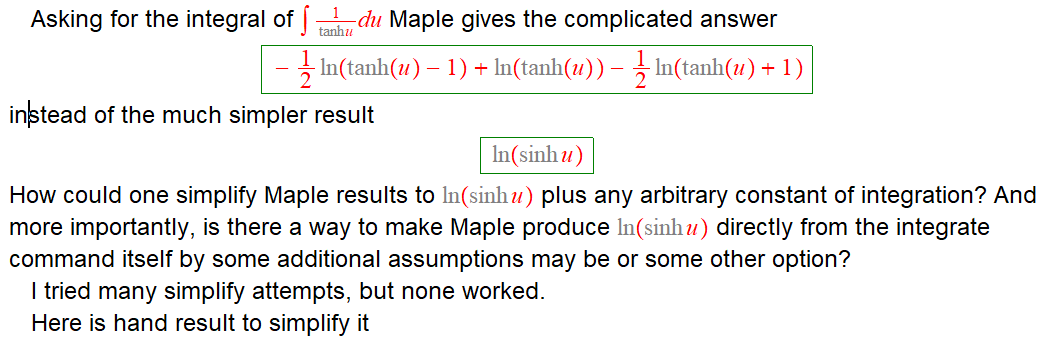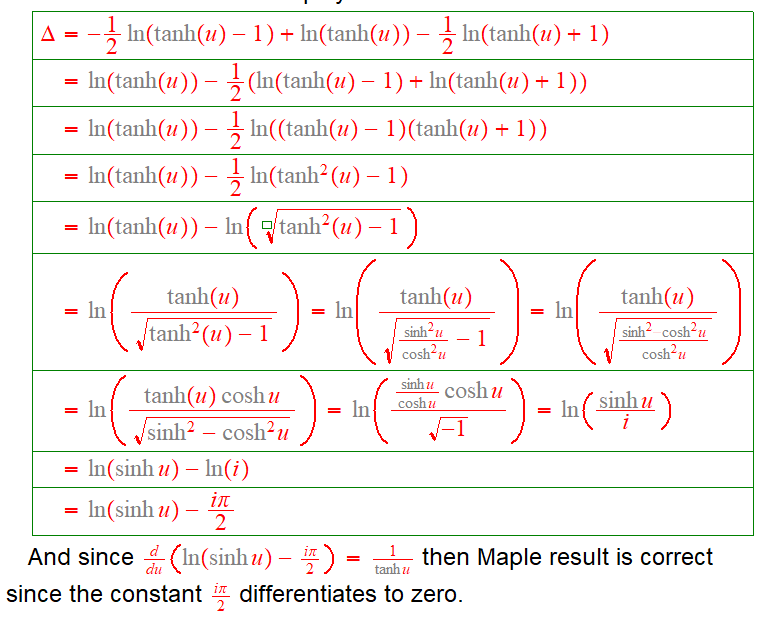This is the Maple code

restart;
result:=int(1/tanh(u),u)

simplify(result,size) ;
simplify(result,symbolic);
simplify(result,ln);
simplify(result,trig);


Any suggestions?

## How to find a rational function with give horizont...

How to find a rational function with give horizontal asymptotes: y=0.7, y=-0.7 and vertical asymptotes: x=0.001, x=-0.001?

## How to partition a list fast? ...

Hello

I have a list with a large number of elements and I need to partition it in chunks of a specific size.  Here is my attempt

listpart := proc(cond::list,nchunk::nonnegint:=1000)
if numelems(cond) < nchunk then
newcond:=[cond]:
else
ss:=[\$(1..numelems(cond))]:
sss:=map(mod,ss,nchunk):
ind:=[ListTools:-SearchAll(0,sss)]:
ind:=[0,op(ind),ifelse(ss[numelems(ss)]=ind[numelems(ind)],[],ss[numelems(ss)])]:
end proc: